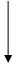Reduction potentials of some ions are given below. Arrange them in decreasing order of oxidising power.

 Ion ${\mathrm{ClO}}_{4}^{-}$ ${\mathrm{IO}}_{4}^{-}$ ${\mathrm{BrO}}_{4}^{-}$ Reduction potential E- /V = 1.19V = 1.65V = 1.74V

Hint: As the reduction potential increases, the oxidising power increases

Greater the SRP value of species higher will be its oxidizing power.
 Species ${\mathbf{E}}_{\mathbf{cell}}^{\mathbf{°}}$ ${\mathrm{ClO}}_{4}^{-}$ ${\mathrm{IO}}_{4}^{-}$ ${\mathrm{BrO}}_{4}^{-}$ 1.19 V 1.65 V 1.74 VSRP of species increases. Oxidizing power increase.

Here, SRP = standard reduction potential.